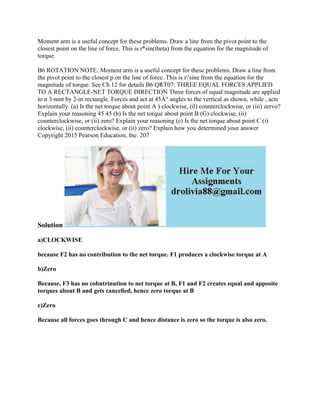Se está descargando tu SlideShare. ×

# Moment arm is a useful concept for these problems- Draw a line from th.docx

Anuncio
Anuncio
Anuncio
Anuncio
Anuncio
Anuncio
Anuncio
Anuncio
Anuncio
Anuncio
AnuncioCargando en…3
×

1 de 2 Anuncio

# Moment arm is a useful concept for these problems- Draw a line from th.docx

Moment arm is a useful concept for these problems. Draw a line from the pivot point to the closest point on the line of force. This is r*sin(theta) from the equation for the magnitude of torque.
B6 ROTATION NOTE: Moment arm is a useful concept for these problems. Draw a line from the pivot point to the closest p on the line of force. This is r\'sine from the equation for the magnitude of torque. See Ch 12 for details B6 QRT07: THREE EQUAL FORCES APPLIED TO A RECTANGLE-NET TORQUE DIRECTION Three forces of equal magnitude are applied to n 3-nmt by 2-m rectangle. Forces and act at 45Â° angles to the vertical as shown, while , acts horizontally. (a) Is the net torque about point A ) clockwise, (il) counterclockwise, or (iii) zervo? Explain your reasoning 45 45 (b) Is the net torque about point B (G) clockwise, (ii) counterclockwise, or (ii) zero? Explain your reasoning (c) Is the net torque about point C (i) clockwise, (ii) counterclockwise, or (ii) zero? Explain how you determined your answer Copyright 2015 Pearson Education, Inc. 207
Solution
a)CLOCKWISE
because F2 has no contribution to the net torque. F1 produces a clockwise torque at A
b)Zero
Because, F3 has no cobntrinution to net torque at B, F1 and F2 creates equal and apposite torques about B and gets cancelled, hence zero torque at B
c)Zero
Because all forces goes through C and hence distance is zero so the torque is also zero.
.

Moment arm is a useful concept for these problems. Draw a line from the pivot point to the closest point on the line of force. This is r*sin(theta) from the equation for the magnitude of torque.
B6 ROTATION NOTE: Moment arm is a useful concept for these problems. Draw a line from the pivot point to the closest p on the line of force. This is r\'sine from the equation for the magnitude of torque. See Ch 12 for details B6 QRT07: THREE EQUAL FORCES APPLIED TO A RECTANGLE-NET TORQUE DIRECTION Three forces of equal magnitude are applied to n 3-nmt by 2-m rectangle. Forces and act at 45Â° angles to the vertical as shown, while , acts horizontally. (a) Is the net torque about point A ) clockwise, (il) counterclockwise, or (iii) zervo? Explain your reasoning 45 45 (b) Is the net torque about point B (G) clockwise, (ii) counterclockwise, or (ii) zero? Explain your reasoning (c) Is the net torque about point C (i) clockwise, (ii) counterclockwise, or (ii) zero? Explain how you determined your answer Copyright 2015 Pearson Education, Inc. 207
Solution
a)CLOCKWISE
because F2 has no contribution to the net torque. F1 produces a clockwise torque at A
b)Zero
Because, F3 has no cobntrinution to net torque at B, F1 and F2 creates equal and apposite torques about B and gets cancelled, hence zero torque at B
c)Zero
Because all forces goes through C and hence distance is zero so the torque is also zero.
.

Anuncio
Anuncio

### Moment arm is a useful concept for these problems- Draw a line from th.docx

1. 1. Moment arm is a useful concept for these problems. Draw a line from the pivot point to the closest point on the line of force. This is r*sin(theta) from the equation for the magnitude of torque. B6 ROTATION NOTE: Moment arm is a useful concept for these problems. Draw a line from the pivot point to the closest p on the line of force. This is r'sine from the equation for the magnitude of torque. See Ch 12 for details B6 QRT07: THREE EQUAL FORCES APPLIED TO A RECTANGLE-NET TORQUE DIRECTION Three forces of equal magnitude are applied to n 3-nmt by 2-m rectangle. Forces and act at 45Â° angles to the vertical as shown, while , acts horizontally. (a) Is the net torque about point A ) clockwise, (il) counterclockwise, or (iii) zervo? Explain your reasoning 45 45 (b) Is the net torque about point B (G) clockwise, (ii) counterclockwise, or (ii) zero? Explain your reasoning (c) Is the net torque about point C (i) clockwise, (ii) counterclockwise, or (ii) zero? Explain how you determined your answer Copyright 2015 Pearson Education, Inc. 207 Solution a)CLOCKWISE because F2 has no contribution to the net torque. F1 produces a clockwise torque at A b)Zero Because, F3 has no cobntrinution to net torque at B, F1 and F2 creates equal and apposite torques about B and gets cancelled, hence zero torque at B c)Zero Because all forces goes through C and hence distance is zero so the torque is also zero.